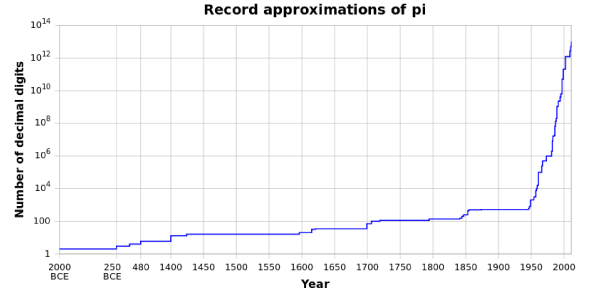How was the value of Pi first calculated? - ProProfs Discuss+ Ask Question

# How was the value of Pi first calculated?Change Image    Delete

Asked by F.William, Last updated: Jan 01, 2021

###Request 0FollowShareAnswer AnonymouslyAnswer LaterCopy LinkT. Moore, Writer, MA, WashingtonIt was Archimedes, the ancient and much revered mathematician who first explained the whole idea of representing the ratio of a circle's circumference to its diameter. This was some 230 years Before Christ.

The ancient Egyptians calculated the area of a circle by a formula that gave the approximate value of 3.1605 for pi. However, it was only in 1700 that mathematicians used the sign - Greek letter - to signify pi.M. Wright

The value of pi was likely first approximated by the ancient Babylonians. One Babylonian tablet estimated to be from around 1680 BC, provided a value for pi of 3.125. The Egyptians, a few year later, also calculated pi to a value of 3.1605. But, the actual first calculation of pi is believed to be done by Archimedes of Syracuse (287-212 BC).

Archimedes used the Pythagorean Theorem to first find the areas of two polygons –one which was drawn inside the circle and one which was drawn outside the circle. Archimedes knew the true value of pi was within the upper and lower bounds of his calculations.Search for Google imagesSelect a recommended image
Upload from your computerCancelSearch for Google imagesSelect a recommended image
Upload from your computerCancelSearch for Google imagesSelect a recommended image
Upload from your computerCancel